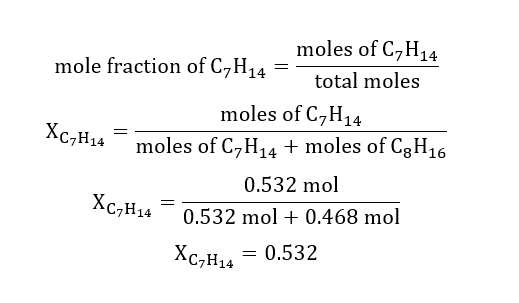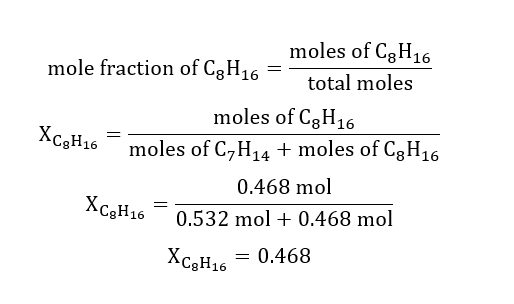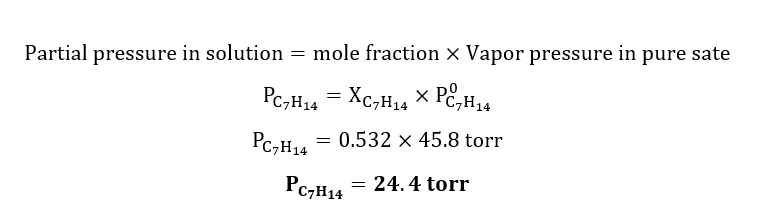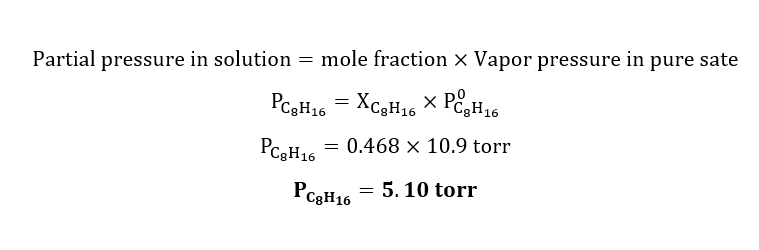# A solution contains of 50.0g (0.532 mol) of heptane, C7H14(l) and 50.0g (0.468 mol) of octane, C8H18(l) at 25°C. At 25°C the vapor pressure of heptane is 45.8 torr, and that of pure octane is 10.9 torr. a) What are the mole fractions of the two components in the solution?b) What are the vapor pressures of each component in the solution?c) What is the total vapor pressure above the solution?

Question
2 views

A solution contains of 50.0g (0.532 mol) of heptane, C7H14(l) and 50.0g (0.468 mol) of octane, C8H18(l) at 25°C. At 25°C the vapor pressure of heptane is 45.8 torr, and that of pure octane is 10.9 torr.

a) What are the mole fractions of the two components in the solution?

b) What are the vapor pressures of each component in the solution?

c) What is the total vapor pressure above the solution?

check_circle

Step 1

Given:

Mass of heptane = 50.0 g

Moles of heptane = 0.532 mol

Mass of Octane = 50.0 g

Moles of Octane = 0.468 mol

vapor pressure of pure heptane = 45.8 torr

vapor pressure of pure octane = 10.9 torr

Step 2: Anwser-a

Calculation for mole fraction of heptane(C7H14):Calculation for mole fraction of Octane(C8H16):Calculation for partial pressure of heptane(C7H14):Calculation for partial pressure of Octane(C8H16):...

### Want to see the full answer?

See Solution

#### Want to see this answer and more?

Solutions are written by subject experts who are available 24/7. Questions are typically answered within 1 hour.*

See Solution
*Response times may vary by subject and question.
Tagged in